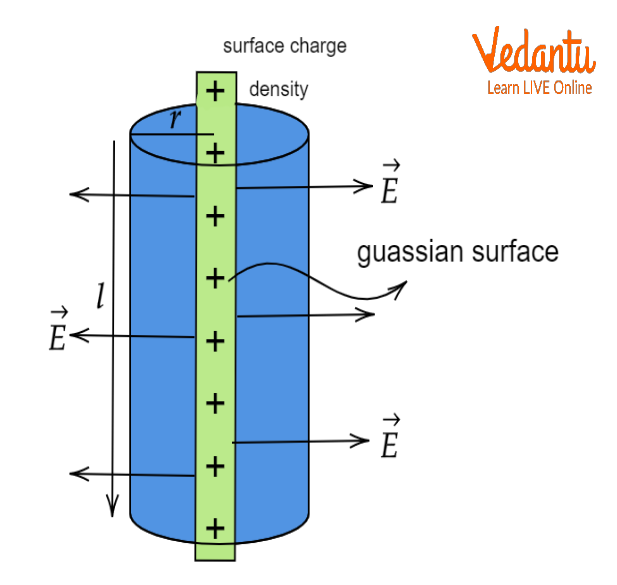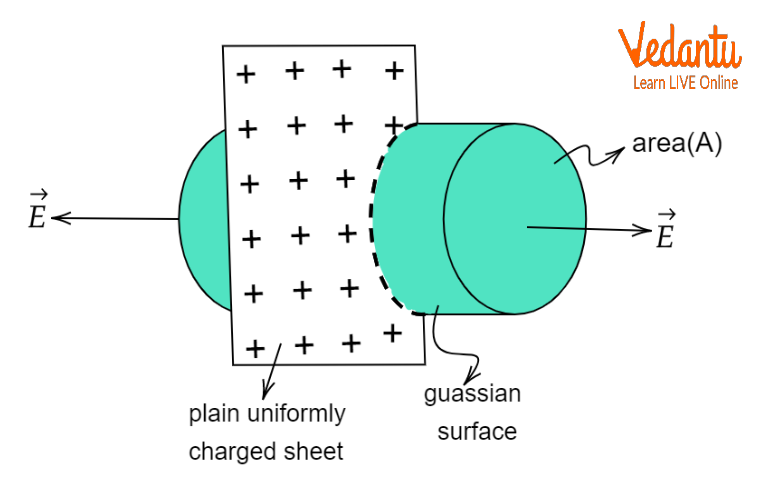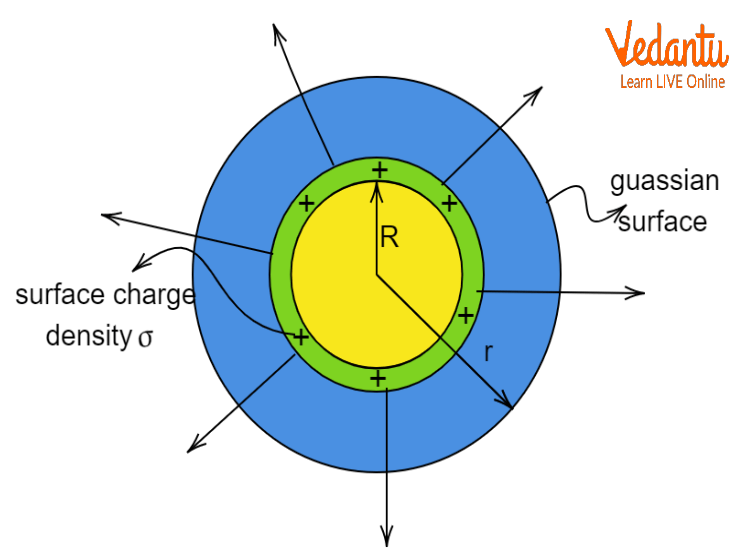Courses
Courses for Kids
Free study material
Offline Centres
More

# Gauss Law - JEE Important TopicLast updated date: 05th Dec 2023
Total views: 22.5k
Views today: 0.22k## What is Gauss Law?

German mathematician and scientist Carl Friedrich Gauss (1777–1855) is credited with creating Gauss' law in the study of electrostatics. He established the relationship between electric flux and the electric charge. The electric flux is the lines of force passing through the electric field. With the help of gauss law we can determine the electric field around various surfaces. The electric flux of any surface can be calculated as the ratio of test charge to the permittivity of free space and this relation was given by Gauss.

In this article, we will study the gauss law in detail with the help of statements and mathematical derivations.

## Gauss Law Formula

The total charge contained within a closed surface, according to the Gauss theorem, is inversely proportional to the total flux contained within the surface. Therefore, if $\phi$ is total flux and ${{\varepsilon }_{0}}$ is electric constant, the total electric charge $Q$ enclosed by the surface is:

$Q=\phi {{\varepsilon }_{0}}$

The Gauss law formula is expressed by;

$\phi =\dfrac{Q}{{{\varepsilon }_{0}}}$

Where,

$Q=$ total charge within the given surface,

${{\varepsilon }_{0}}=$ the electric constant.

## The Gauss Theorem

The net flux through a closed surface is directly proportional to the net charge in the volume enclosed by the closed surface.

$\phi =E\cdot dS =\dfrac{{{q}_{net}}}{{{\varepsilon }_{0}}}$

In simple words, the Gauss theorem relates the ‘flow’ of electric field lines (flux) to the charges within the enclosed surface. The net electric flow is always zero if no charges are contained by a surface. Accordingly, the number of electric field lines entering the surface equals the number of field lines ejecting from it.

The Gauss theorem statement also gives an important corollary:

• Any closed surface's electric flux is exclusively produced by the sources (positive charges) and sinks (negative charges) of the electric fields it encloses.

• The electric flow is unaffected by any charges that are present below the surface. Additionally, the sources and sinks of electric fields can only be electric charges. For instance, shifting magnetic fields are unable to serve as sources or sinks of electric fields.

• Given that the surface on the left contains a net charge, its net flux is not zero. Since the surface on the right does not enclose any charges, the net flux is zero.

## Gauss Law Derivation

Derivation of Gauss' law that applies only to a point charge

We start by formulating a special case of Gauss' law that only holds true in the case of a point charge, which we assume to be positive. A positive point charge's electric field lines extend in all directions from the charge.

$E=\dfrac{q}{4\pi {{\varepsilon }_{0}}{{r}^{2}}}$

The magnitude $E$ of the electric field at a distance r from the charge $+q$ is $E=kq/{{r}^{2}}$. The constant $k$ can be expressed as $k=1/4\pi {{\varepsilon }_{0}}$ , where ${{\varepsilon }_{0}}$ is the permittivity of free space.

With this substitution, the magnitude of the electric field becomes

$E=\dfrac{q}{4\pi {{\varepsilon }_{0}}{{r}^{2}}}$.

Now let's position this positive point charge at the centre of a hypothetical sphere with radius r. Although it's not always necessary, a closed surface like this is referred to as a Gaussian surface.

Everywhere on the surface, the electric field is of the same magnitude and is perpendicular to the surface.

The surface area $A$ of a sphere is $4\pi r^{2}$ , and the magnitude of the electric field can be written in terms of this area as $E=\dfrac{q}{A{{\varepsilon }_{0}}}$.

When the electric field is perpendicular to the surface, Gauss' law for a point charge can be expressed primarily as $EA=\dfrac{q}{{{\varepsilon }_{0}}}$, where the left side of equation is the product of the magnitude $E$ of the electric field at any point on the Gaussian surface and the area $A$ of the surface.

In Gauss' law, this product is especially important and is called the electric flux and we can write as ${{\phi }_{E}}=E\cdot A=EAcos\theta$.

In other words, the scalar product of $A$ and $E$ is used to determine the electric flux.

Here, $\theta$ is the angle between the electric field and the area vector. Note that the area vector is normal to the surface.

This implies that the electric field becomes parallel to the area vector when it is perpendicular to the surface. Thus,

$\phi _{E}=EA~cos~0=EA$

Using both equations we now can write the equation of electric flux for a point charge:

${{\phi }_{E}}=\dfrac{q}{{{\varepsilon }_{0}}}$

This result indicates that, aside from the constant $\varepsilon _{0}$, the electric flux $\phi _{E}$ depends only on the charge $q$ within the Gaussian surface and is independent of the radius r of the surface.

## Applications of Gauss Law

Gauss's law is mostly used to determine the electric field caused by:

An infinitely charged uniform straight wireInfinitely Charged Uniform Straight Wire

Electric field is given as:

$E=\dfrac{\lambda }{2\pi {{\varepsilon }_{o}}r}$

A uniformly charged infinite plane sheet:Uniformly Charged Infinite Plane Sheet

The electric field due to infinite plane sheet is given as:

$E=\dfrac{\sigma }{2{{\varepsilon }_{o}}}$

A uniformly charged thin spherical shell: There are two ways to assess the electric field caused by the spherical shell: Outside and inside of the spherical shell, respectively, of the electric field.Uniformly Charged Thin Spherical Shell

• Outside Spherical Shell: Electric Field is given as:

$E={}^{kq}/{}_{{{r}^{2}}}$

• Inside Spherical Shell: Because there is no enclosed charge, there is no electric field inside the spherical shell.

• The electrical charge that is present in or enclosed by a closed surface is explained by Gauss's law.

• The total flux linked with a closed surface is $\dfrac{1}{{{\varepsilon }_{0}}}$ times the charge enclosed by the closed surface is known as Gauss Law.

• Complex electrostatic problems requiring unusual symmetries like cylindrical, spherical, or planar symmetry can be resolved using Gauss's Law.

## Conclusion

According to Gauss's law, the electric charge enclosed in any closed surface is directly proportional to the total quantity of electric flux travelling through it. The law that explains the relationship between electric charge distributions and the resulting electric field is referred to as Gauss Law, also known as Gauss's flow theorem or Gauss's theorem. Coulomb's law is used to compute the electrical field of a surface, but the Gauss law is needed to determine how the electrical field is distributed on a closed surface. It clarifies the electrical charge contained in the closed or present in the closed surface.

## FAQs on Gauss Law - JEE Important Topic

1. What is the importance of the Gaussian surface? State two properties of the Gaussian surface.

A Gaussian surface is a contained surface in three dimensions used to determine the flux of a vector field (gravitational field, the electric field, or magnetic field.) Because of the symmetric charge distribution, the Gaussian surface aids in determining the strength of the electric field.

Essential characteristics of a Gaussian surface include the following:

• It must be a closed surface so that the locations of points inside, on, and outside the surface can be distinguished clearly.

• The area through which the electric field must be calculated must be traversed by this surface.

2. Is it possible to apply Gauss's law to a non-uniform electric field?

Regardless of whether an electric field is uniform or not, Gauss's law holds true for all electric fields. When the field is symmetrical, such as the field surrounding a uniform spherical charge distribution, it becomes easier to calculate the electric field intensity using Gauss's law. The electric field which is present near the  infinite plate has uniform charge distribution. Although Gauss rule is applicable for all electric fields, manual calculation is challenging when the electric field is not symmetrical and uniform.